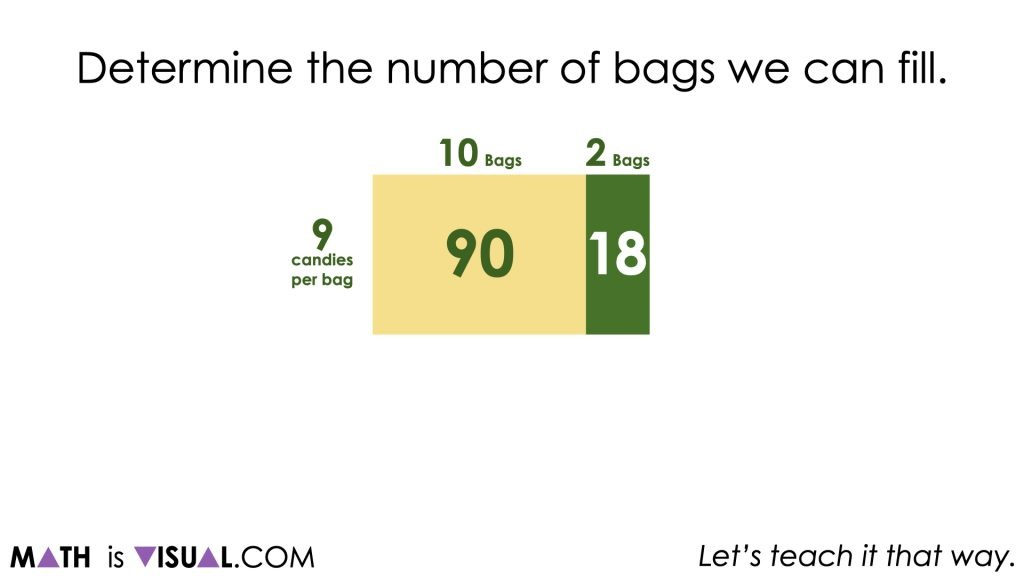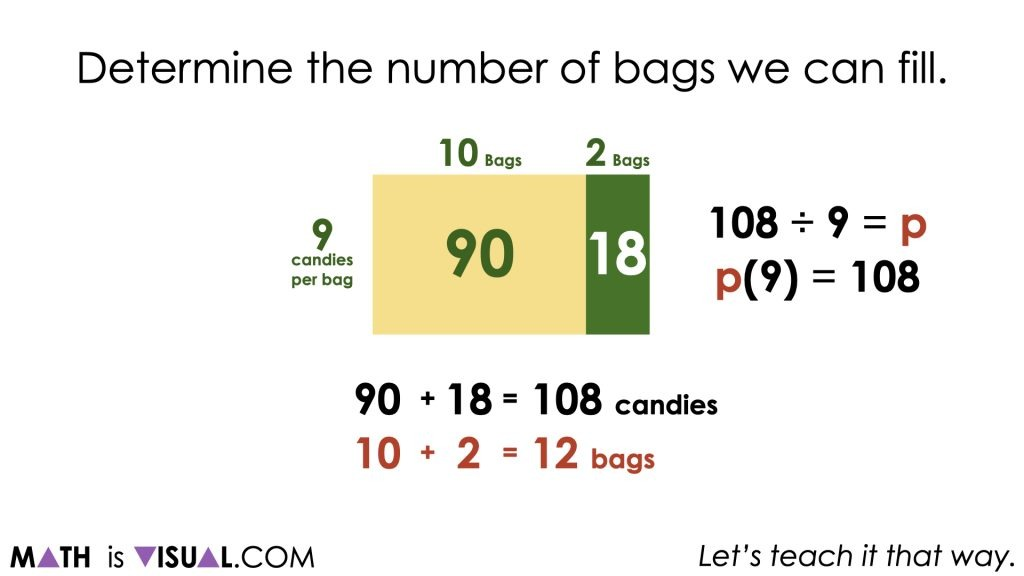# Using Division to Solve One-Step Equations – Part 2

Visual math talk prompts highlighting the relationship between multiplication and quotative division to solve one-step algebraic equations.

## In This Set of Math Visual Prompts…

Students will explore quotative division problems with context and connect them to one-step algebraic equations.

## Intentionality…

This set of visual math talk prompts is taken from the Math Talk section of Day 4 in the Make Math Moments Problem Based Unit called Sowing Seeds Revisited. The purpose of the Math Talk is to reinforce key concepts and big ideas from this problem based math unit including:

• There are two types of division;
• Quotative division is when the total quota is known (the dividend), and the number per group or the rate (the divisor) is known;
• Quotative division reveals the number of copies or iterations of a rate that can be derived from the overall quota (the dividend);
• In quotative division, the dividend and the divisor have the same unit;
• The dividend from any division sentence can be decomposed into smaller parts to allow for friendlier division by the divisor. This strategy is known as partial quotients. (i.e.: 85 ÷ 5 = 45 ÷ 5 + 40 ÷ 5 = 9 + 8 = 17);
• Division is the inverse operation of multiplication;
• Variables are used to represent changing or unknown quantities;
• When solving an equation where the coefficient of the unknown variable is not equal to 1, division is required to determine the value of the unknown variable and the context of the problem determines which type of division is required.

Unsure about some of the terminology listed above?

## Preparing to Facilitate

Present the following multiplication and division equations one at a time. Encourage students to describe a quotative context that could be represented by each of the division sentences such as kilograms of bird seed (dividend) and kilograms per feeder (divisor) like we did in the last lesson.  Students should use a model of their choice to solve and/or represent their thinking.

108 ÷ 9 = p

p(9) = 108

Two (2) additional prompts are shared in the Teacher Guide from Day 4 of the Sowing Seeds Revisited problem based math unit

Consider using the visual math talk prompts to help you prepare to facilitate this math talk or to assist you in doing so in a visual fashion. The purpose of this math talk is to reveal that both the division and one-step algebraic equation can be solved using the same strategy and model.

## Visual Math Talk Prompt #1

In this visual math talk prompt we begin with a low floor quotative division problem asking students:

108 candies are divided into bags with 9 candies in each bag.

How many bags can we fill?

Although students are free to leverage the strategy and mathematical model of their choice, we are hoping to elicit the use of the open array or area model to demonstrate partial products and/or partial quotients.In the visual example, we can see that the dividend was decomposed into 90 and 18 to more easily come to the result for our quotient.In the visual example, we can see that the dividend was decomposed into 90 and 18 to more easily come to the result for our quotient.

## Want to Explore These Concepts & Skills Further?

Two (2) additional math talk prompts are available in Day 4 of the Sowing Seeds Revisited problem based math unit that you can dive into now.

Why not start from the beginning of this contextual 5-day unit of ratios and rates real world lessons from the Make Math Moments Problem Based Units page.

Did you use this in your classroom or at home? How’d it go? Post in the comments!

Math IS Visual. Let’s teach it that way.## Using Division to Solve One-Step EquationsREGISTER FOR OUR FREE WEBINAR:
Reaching Every Student After The Toughest Year Ever
How to Focus On The Concept Holding Your Students Back

WATCH THE REPLAY NOW